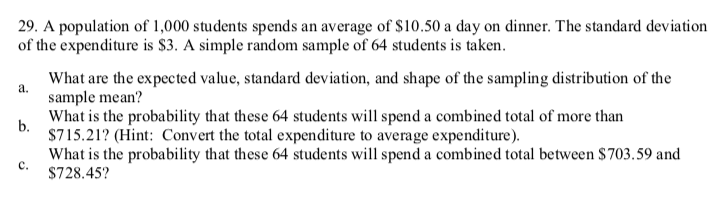# 29. A population of 1,000 students spends an average of \$10.50 a day on dinner. The standard deviationof the expenditure is \$3. A simple random sample of 64 students is taken.What are the expected value, standard deviation, and shape of the sampling distribution of thea.sample mean?What is the probability that these 64 students will spend a combined total of more thanb.\$715.21? (Hint: Convert the total expenditure to average expenditure).What is the probability that these 64 students will spend a combined total between \$703.59 andc.\$728.45?

Question
319 viewshelp_outlineImage Transcriptionclose29. A population of 1,000 students spends an average of \$10.50 a day on dinner. The standard deviation of the expenditure is \$3. A simple random sample of 64 students is taken. What are the expected value, standard deviation, and shape of the sampling distribution of the a. sample mean? What is the probability that these 64 students will spend a combined total of more than b. \$715.21? (Hint: Convert the total expenditure to average expenditure). What is the probability that these 64 students will spend a combined total between \$703.59 and c. \$728.45? fullscreen
check_circle

Step 1

29.

c.

The probability that these 64 students will spend a combined total between\$703.59 and \$728.45.

From the given information, the value of average is \$10.50, standard deviation is \$3 and sample size (n) is 64.

By the central limit theorem, the z-score formula is given below:

Step 2

Since n > 30 the sampling distribution is approximately normal.

The value of sample mean for 703.59 is,

Mean  = sum of the observation / sample size

= 703.59/ 64

...

### Want to see the full answer?

See Solution

#### Want to see this answer and more?

Solutions are written by subject experts who are available 24/7. Questions are typically answered within 1 hour.*

See Solution
*Response times may vary by subject and question.
Tagged in
MathStatistics

### Other